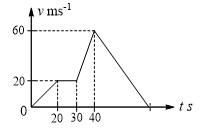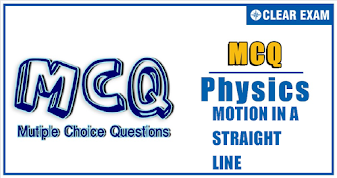## Physics Quiz-9

If an object changes its position with respect to its surroundings with time, then it is called in motion. It is a change in the position of an object over time. Motion in a straight line is nothing but linear motion. As the name suggests, it’s in a particular straight line, thus it can be said that it uses only one dimension.

Q1. Four persons are initially at the four corners of a square whose side is equal to d. Each person now moves with a uniform speed V in such a way that the first moves directly towards the second, the second directly towards the third, the third directly towards the fourth, and the fourth directly towards the first. The four persons will meet after a time equal to
•  d/V
•  2d/3V
•  2d/√3  V
•  d/√3  V
Solution
From considerations of symmetry, the four persons meet at the centre of the square. The displacement from the corner to the centre of the square for each person is give by
S_r=√(d^2+d^2 )/2=d/√2
The speed of each person can be resolved into two components: the radial component and the perpendicular component. Throughout the journey, the radial component of velocity towards the centre is given by
V_r=V cos⁡〖45°=V/√2,〗 Time =S_r/V_r =(d/√2)/(V/√2)=d/V

Q2.The displacement-time graph of a moving particle is shown in Fig. The instantaneous velocity of the particle is negative at the point

•  D
•  F
•  C
•  E
Solution
The slope of the graph is negative at this point.

Q3. The velocity-time graph of a body is shown in Fig. The ratio of average acceleration during the intervals OA and AB is

•  1
•  1/2
•  1/3
•  3
Solution
During OA, acceleration =tan⁡〖30°=1/√3  ms^(-2) 〗
During AB, acceleration =-tan⁡〖60°=-√3〗  ms^(-2)
Required ratio =(1/√3)/√3=1/3

Q4. A body travels 200 cm in the first 2 s and 220 cm in the next 4 s with deceleration. The velocity of the body at the end of the 7th second is
•  5 cm/s
•  10 cm/s
•  15 cm/s
•  20 cm/s
Solution
200=u×2-(1/2)a(2)^2
or u-a=100    …(i)
200+220=u(2+4)-(1/2) (2+4)^2 a
or  u-3a=70   …(ii)
Solving Eqs. (i) and (ii), we get a=15 cms^(-2) and u=115 cms^(-1)
Further, v=u-at=115-15×7=10 cms^(-1)

Q5.The relation between time t and distance x is
t=αx^2+βx
Where α and β are constant. The retardation is
•  2αv^3
•  2βv^3
•  2αβv^3
•  2β^2 v^3
Solution
At=αx^2+βx
Differentiating: 1=2α dx/dt.x+β dx/dt
v=dx/dt=1/(β+2αx);dv/dt=(-2αv)/(β+2αx)^2 =-2αv^3

Q6.
 The velocity-time graph of a body is given in Fig. The maximum acceleration in ms^(-2) is•  4
•  3
•  2
•  1
Solution
Maximum acceleration will be from 30 to 40 s, because slope in this interval is maximum
a=(v_2-v_1)/(t_2-t_1 )=(60-20)/(40-30)=4 ms^(-2)

Q7.
The velocity-time graph of a body is shown in Fig. The displacement of the body in 8 s is

•  9 m
•  12 m
•  10 m
•  28 m
Solution
Displacement = area under graph
=2×2+1/2(2+6)×1+1/2×1×6-1/2×1×6-1×6+2×4
=10 m

Q8. A particle moves along the x-axis in such a way that its position coordinate (x) varies with time (t) according to the expression x=2-5t+6t^2. Its initial velocity is
•  -3 ms^(-1)
•  -5 ms^(-1)
•  2 ms^(-1)
•  3 ms^(-1)
Solution
x=2-5t+6t^2  ⇒v=dx/dt=-5+12t
v_(t=0)=-5+12×0=-5 ms^(-1)

Q9.
 A particle is moving along the x-axis whose instantaneous speed is given by v^2=108-9x^2. The acceleration of the particle is

•  -9x ms^(-2)
•  -18x ms^(-2)
•  (-9x)/2  ms^(-2)
•  None of these
Solution
v^2=108-9x^2
a=dv/dt=dv/dx∙dx/dt=d(√(108-9x^2 ))/dx∙dx/dt
a=(1(-18x))/(2√(108-9x^2 ))∙√(108-9x^2 )=-9x ms^(-2)
Alternative: Differentiating w.r.t. x, we get
2v dv/dx=-18x
⇒vdv/dx=-9x ⇒a=-9x    (∵v dv/dx=a)
Q10. If x denotes displacement in time t and x=a cos⁡ t, then acceleration is
•  a cos⁡ t
• -a cos⁡ t
•  a sin⁡ t
• -a sin ⁡t
Solution
x=a cos⁡〖t,dx/dt=-a sin⁡t;(d^2 x)/(dt^2 )=-a cos⁡t 〗#### Written by: AUTHORNAME

AUTHORDESCRIPTION## Want to know more

Please fill in the details below:

## Latest NEET Articles\$type=three\$c=3\$author=hide\$comment=hide\$rm=hide\$date=hide\$snippet=hide

Name

ltr
item
BEST NEET COACHING CENTER | BEST IIT JEE COACHING INSTITUTE | BEST NEET & IIT JEE COACHING: Motion In a Straight Line-Quiz-9
Motion In a Straight Line-Quiz-9
https://1.bp.blogspot.com/-_KLB3j4_t04/YN796ZrnaJI/AAAAAAAAQV0/6p5Bk2YWE0Qp2o09-L6Sq_jMUsUSTTwJwCLcBGAsYHQ/w337-h178/QUIZ%2BBACK.png
https://1.bp.blogspot.com/-_KLB3j4_t04/YN796ZrnaJI/AAAAAAAAQV0/6p5Bk2YWE0Qp2o09-L6Sq_jMUsUSTTwJwCLcBGAsYHQ/s72-w337-c-h178/QUIZ%2BBACK.png
BEST NEET COACHING CENTER | BEST IIT JEE COACHING INSTITUTE | BEST NEET & IIT JEE COACHING
https://www.cleariitmedical.com/2021/07/motion-in-straight-line-quiz-9.html
https://www.cleariitmedical.com/
https://www.cleariitmedical.com/
https://www.cleariitmedical.com/2021/07/motion-in-straight-line-quiz-9.html
true
7783647550433378923
UTF-8

STAY CONNECTED